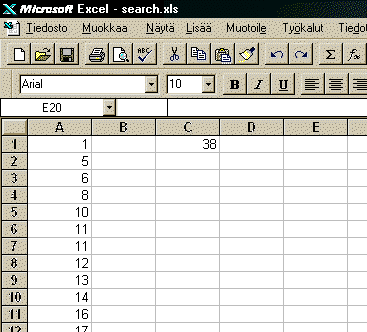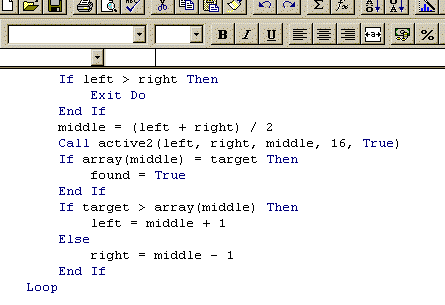Computer Science Visualizations

Spreadsheet programs like Microsoft Excel are generally considered only as tools for accounting and budgeting. This limited view restricts their use, though they offer facilities to visualize, illustrate, and describe different phenomena, and experiment with these. In Excel this expressive power has been combined with a friendly user-interface. Therefore Excel offers a light, adaptive, and inspiring platform for creating visualizations or various needs also in Computer Science. A teacher can prepare visualizations for teaching, or visualizations can be student assignments. We demonstrate eight Computer Science visualizations.

Bubble sort. While being one of the simplest sorting algorithms, bubble sort is often used as an example in basic programming courses. This demonstration uses the chart tool of Excel to visualize the changes in an array while applying the algorithm.

Binary search. Binary search is one of the most efficient search methods (although it requires the data to be sorted). Our demonstration visualizes the binary search algorithm while searching for a value given by a user from an array. (The demonstration is explained in detail below.)

Editing distance. Editing distance describes the number of basic editing operations required to convert a string into another. It can be computed with a method called dynamic programming. Our demonstration uses 3-dimensional chart to illustrate this process.

Factorial. This demonstration illustrates the use of a stack data structure and recursion to determine a factorial of a given number.

Huffman code generation. Huffman code is used in data compression. The idea is to give frequent characters (or values) in the data to be compressed shorter codes.

Pairwise nearest neighbors. This demonstration visualizes how to create clusters from a given set of vectors. this algorithm is applied in image compression.

Hashing. Hashing is a method to store data so that it can be retrieved quickly. Each data unit has a key, which is used with a hash function to locate the data unit. We present a visualization of a closed hashing algorithm.

TSP. The Euclidean Travelling Salesman Problem can be solved approximately by the k-exchange technique. We present a simple visualization of 2-exchanges.

Algorithm Animation with Excel

We have studied how to make quickly adaptive animations of algorithms with the Microsoft Excel spreadsheet program. Two standard features of Excel, i.e. data visualization and macro programming, provide together an easy-to-use environment to animate algorithms.

All the phases of our approach are easy to carry out for even a novice in Computer Science familiar with the basics of Excel. Our approach is ideal for course assignments in many areas of Computer Science, and it can be applied even in research.

In its flexibility, the Excel approach to algorithm animation can be compared to Polaroid cameras: while the quality might not be the most refined, the quick results are practical even for unexpected purposes. And as Polaroid cameras, it is everyman's application: it works wherever Excel does.

An Example: Binary Search

Here is an example in detail to clarify our ideas. Binary search is a well-known method for searching for an item in a sorted array. In the beginning the search range is the whole array. Then the search key is compared with the middle element of the range. If they are equal, the wanted item has been found. If the key is smaller, the same procedure is applied recursively to the left half of the search range, otherwise to the right half.

There are three phases of creating an animation with Excel:

Phase 1: Put data structures to the worksheetTo animate this search algorithm, a chart which represents the array has to be formed. Let us assume that the array has 30 elements with non-decreasing values. The values are inserted in a normal Excel sheet to cells A1 through A30.

Phase 2: Make a chart which visualizes the data structuresThen this range is selected and the chart wizard of Excel is applied to create a bar chart. Other chart types are also possible.

Phase 3: Write codeBesides forming a chart, the binary search algorithm is written in Visual Basic. The search and animation code is shown in Program 1.

Sub bin_search()
Dim found As Boolean
Dim a(30), target, left, right, mid As Integer
target = Range("C1").Value
found = False
left = 1
right = 30
Do Until found
If left > right Then
Exit Do
End If
mid = (left + right) / 2
call colorize(left,right,mid,16,true)
If a(mid) = target Then
found = True
ElseIf target > a(mid) Then
left = mid + 1
Else
right = mid - 1
End If
Loop

If found Then
Call Colorize(mid, mid, 0, 10, true)
End If
End Sub
Program 1.
The algorithm does not animate itself, and so the chart manipulation commands are inserted in the code. The animation have to be shown as color changes, which are defined in Program 2.
Sub Colorize(ByVal start As Integer, ByVal end As Integer, ByVal middle as Integer, color As Integer, clear as boolean)
If clear Then
ActiveSheet.ChartObjects("chart1").
Chart.SeriesCollection(1).Interior.
ColorIndex = 6
End If

For i = start To end
If i = middle Then
ActiveSheet.ChartObjects("chart 1").
Chart.SeriesCollection(1).
Points(i).Interior.ColorIndex = 3
Else
ActiveSheet.ChartObjects("chart 1").
Chart.SeriesCollection(1).
Points(i).Interior.ColorIndex = color
End If
Next
End Sub
Program 2.
The search range is shown with dark bars and the found value, if any, is shown with a red bar. A screenshot of animation is shown in Figure 1 where 38 is the search key.